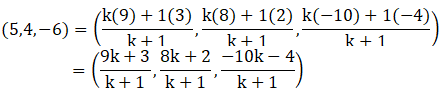If the points A(3, 2, -4), B(9, 8, -10) and C(5, 4, -6) are collinear, find the ratio in which C divided AB.

Asked by Sakshi | 1 year ago |  129

##### Solution :-

Given:

The points A (3, 2, -4), B (9, 8, -10) and C (5, 4, -6)

Let C divides AB in ratio k: 1

Three points are collinear if the value of k is the same for x, y and z coordinates.

Then, m = k and n = 1

A(3, 2, -4), B(9, 8, -10) and C(5, 4, -6)

Coordinates of C are:On comparing we get,

$$\dfrac{ [9k + 3] }{[k + 1]}$$ = 5

9k + 3 = 5(k + 1)

9k + 3 = 5k + 5

9k – 5k = 5 – 3

4k = 2

k = $$\dfrac{2}{4}$$

$$\dfrac{1}{2}$$

$$\dfrac{ [8k + 2] }{ [k + 1]}$$ = 4

8k + 2 = 4(k + 1)

8k + 2 = 4k + 4

8k – 4k = 4 – 2

4k = 2

k = $$\dfrac{2}{4}$$

$$\dfrac{1}{2}$$

$$\dfrac{ [-10k – 4] }{[k + 1] }$$= -6

-10k – 4 = -6(k + 1)

-10k – 4 = -6k – 6

-10k + 6k = 4 – 6

-4k = -2

k = $$\dfrac{-2}{-4}$$

$$\dfrac{1}{2}$$

The value of k is the same in all three cases.

So, A, B and C are collinear [as, k = $$\dfrac{1}{2}$$]

We can say that, C divides AB externally in ratio 1:2

Answered by Aaryan | 1 year ago

### Related Questions

#### A(1, 2, 3), B(0, 4, 1), C(-1, -1, -3) are the vertices of a triangle ABC. Find the point in which the bisector of the

A(1, 2, 3), B(0, 4, 1), C(-1, -1, -3) are the vertices of a triangle ABC. Find the point in which the bisector of the angle ∠BAC meets BC.

#### The mid-points of the sides of a triangle ABC are given by (-2, 3, 5), (4, -1, 7) and (6, 5, 3). Find the coordinates

The mid-points of the sides of a triangle ABC are given by (-2, 3, 5), (4, -1, 7) and (6, 5, 3). Find the coordinates of A, B and C.

#### Find the ratio in which the line segment joining the points (2, -1, 3) and (-1, 2, 1) is divided by the plane

Find the ratio in which the line segment joining the points (2, -1, 3) and (-1, 2, 1) is divided by the plane x + y + z = 5.